# Over 10 Actionable VLOOKUP Examples Explained

As we all know, the best way to learn something is to get your hand on it, make mistakes and learn from them.

As they say, practice makes a man perfect.

That’s what this Excel VLOOKUP Example guide is all about.

I believe that a well-thought-out example explains a concept much better than a description of the underlying theory.

Instead of explaining to you a painful review of every aspect of the VLOOKUP function, I personally selected and explained 10+ examples of the VLOOKUP function.

These examples will help you learn how the function works and how it can be used in a gazillion variety of situations.

You will walk through examples that solve practical problems while furthering your knowledge of Excel as a whole.

So, if you want to see the best VLOOKUP examples, then you’ll love this guide.

## VLOOKUP EXAMPLE 1: Commission Calculator

Many sales jobs pay workers on a commission basis. The commission of such workers is often calculated as a percentage of the sales they made.

The more sales they make, the more commission they earn, and the more money the company makes too.

The commission can be set on flat-rate, which is usually a percentage, say 7 percent, on any sales the representative makes.

Businesses can also use progressive commission, where the percentage of commission rises as sales rep hit certain targets.

For example, selling \$10,000 worth of goods or services can earn the representative 10 percent commission, a 15 percent commission on the next \$10,000, and so on.

The example below shows a worksheet that uses the VLOOKUP function to determine the commission a sales rep gets, based on how much sales she makes.

See screenshot: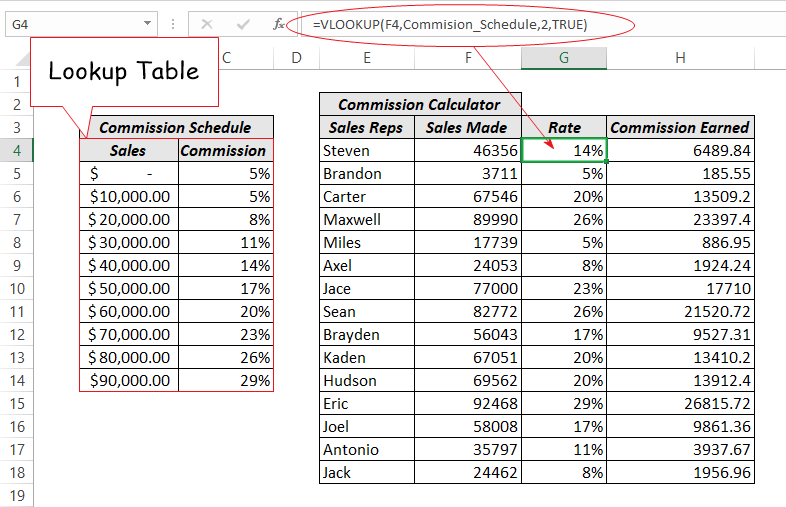This particular VLOOKUP example is called “range lookup”. This means that the formula is not out looking for an exact match, but for a match that falls within a range of values (approximate match).

For instance, just as seen in the above table, the VLOOKUP formula retrieved 5% for any value that falls below 20,000 and 8% for any value that falls between 20,000 and 30,000, and so on.

### VLOOKUP Formula Analysis

Let’s throw more light on the formula used in the above example.

=VLOOKUP(F4,Commision_Schedule,2,TRUE)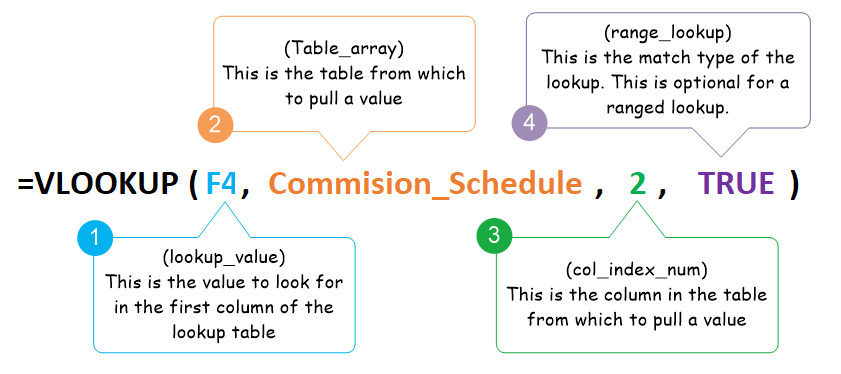Actually, the picture said it all.

In the formula;

• The first argument referred to the cell that contains the value to look for. (That is Cell F4).
• The second argument is also referring to the table from which to pull the commission rate. (That is the Commision_Schedule table). The table array argument can either be a named range or just a normal range. For instance, using B4:C14 is the same range as the commision_Schedule. However, if you still want to use a normal range and not a named range, ensure to make it an absolute reference – like this: \$B\$4:\$C\$14.
• The third argument specifies the column in the table from which to pull the commission rate. (That is column 2 of the lookup table).
• The final argument is set to TRUE which means “approximate match”. VLOOKUP approximate match will search for the largest value that is less than the lookup value. For instance, in the example above, the formula in cell G4 searched to find \$46,356 in the first column of the lookup table. And since the table doesn’t have an exact match for this search, it retrieved the commission rate for the largest value that is less than the lookup value, (which is \$40,000).

After you finished writing the formula in the first cell, you can use the fill handle to copy the formula to the rest of the cells.

See screenshot:## VLOOKUP EXAMPLE 2: Income Tax rate Calculator

This particular example will make more sense to you if you have some accounting knowledge (Taxation to be precise). If you are one of those who hate accounting, you are welcome to skip to the next example.

In this VLOOKUP example, we are going to write a formula that returns the tax rate for various income levels.

Excel tax rate calculator is another good candidate for the VLOOKUP function (range lookup to be specific).

In accounting, there is this tax system called progressive system of taxation where the income of individuals and business follow an accelerating schedule.

This means that the higher your income, the higher the percentage of tax you pay, and the lower your income, the lower the percentage of tax you pay.

In fact, if you earn a very little income, you pay no income tax at all.

We call it Tax Brackets, the division at which tax rate change as the taxable income increase or decrease.

The following tax schedule said it all:

See screenshot: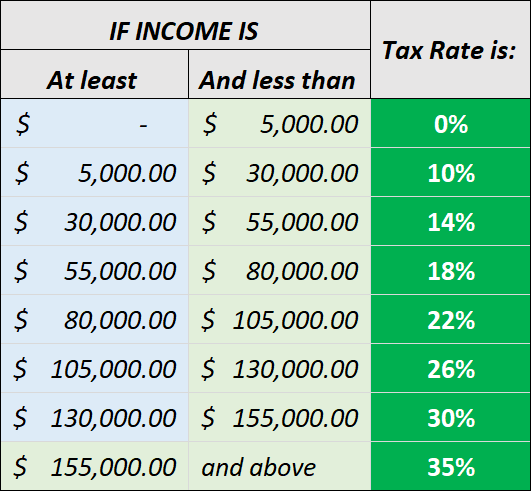The above schedule is a very clear example of the progressive tax system.

As you can see, the low-income earners who earns less than \$5,000 has no obligation to pay tax on their income whatsoever.

You are only qualified for tax as soon as your income increase up to \$5,000 and above, but with a minimal tax rate as a beginner.

Each penny or dollar you earn put you into a tax bracket or category, resulting into a higher tax rate once your taxable income enters a new tax bracket or category.

Now the goal of this Excel VLOOKUP example is to write a formula that will retrieve the tax rate of any specified salary.

See screenshot: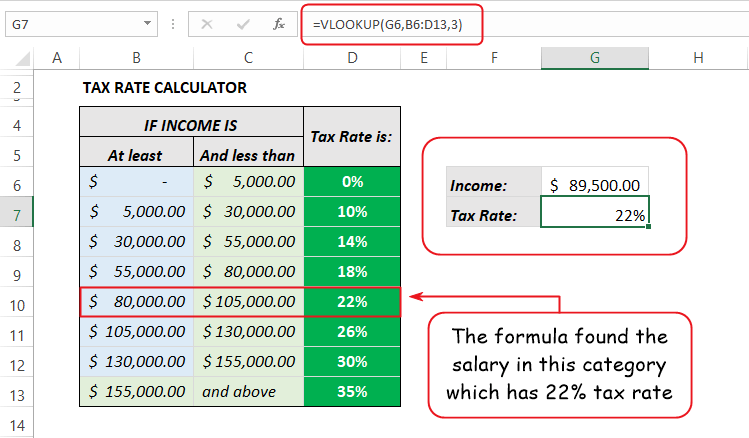As seen in the above worksheet, the lookup table is B6:D13, and the lookup value is in cell G6, which contain the taxable income.

If this formula was looking for an exact match, it would have encounter an error since the salary amount in cell G6 is nowhere to be found in the first column of the lookup table.

But here, the formula performs a range lookup where it is not looking for an exact match, but for a match that falls within a range of values – in this case, between \$80,000 and \$104,000.

As soon as it found the category of the salary, it returned the tax rate for it.

Remember: The formula did not retrieve the tax rate base on the first two columns, it does that only by looking in the first column of the lookup table. The second or middle column is just a helper column to help you understand the various tax brackets or categories.

Now let’s look at the analysis.

### VLOOKUP Formula Analysis

Below is the formula used to perform the task in this example:

=VLOOKUP(G6,B6:D13,3)• The first argument in this formula is referring to the cell that contains the taxable income we are looking for. (That is Cell G6).
• The second argument also is referring to the table from which to pull the tax rate. (That is cell B6:D13).
• The third argument specifies the column in the table from which to pull the tax rate. (That is column 3 of the lookup table).

But wait! VLOOKUP has four arguments, but this formula uses only three of them and yet did not encounter any error.

This is because the last argument is optional when you are dealing with a “range lookup” (approximate match).

By default, VLOOKUP goes with an approximate match. So, when you leave out the final argument, VLOOKUP will assume that you accept to ride with its default match, which is the approximate match.

But if you still want to always include the match type, specify TRUE or 1 for this argument.

With the approximate match, if the value you are looking for is not found in the lookup column, Excel will not border you with the familiar #N/A error message. Instead, it’ll use the highest value that is lower than the specified value.

Refer to this article for more notes on the VLOOKUP match type.

## EXAMPLE 3: Return products and prices using ID

Have you ever worked with any good accounting software?

If yes, then you are fully aware of how effortless producing invoices can be.

The products along with their prices are fully integrated in an interesting way.

As soon as you enter a product ID, the name and price for that product automatically show without you typing them yourself.

You can also design such a system in Excel using the VLOOKUP function.

See What I’m talking about in the following illustration:This example contains two versions of the VLOOKUP function in both the product and price columns.

As for the amount column, it doesn’t contain a VLOOKUP formula though. It only multiplies the quantity by the price to get the total amount.

That being said, our goal is to write two formulas that will return the product name and its price when a product ID is specified.

Know that: in the past two examples, we performed ranged lookups by using the approximate match. However, in this example, our goal is to find exact matches for our search terms. So, this an exact match VLOOKUP example.

Take a look in the following worksheet:The above worksheet is in show formula mode. In this mode, Excel displays formulas instead of the resulting values.

In the following analysis, let’s have a pragmatic analysis of the two formulas.

### VLOOKUP example 3 Formula Analysis

As seen in the worksheet, in the products column, the lookup table is B4:D14 which was renamed to ProductList, and the lookup value argument is referring to the corresponding cells in the ID column, which contain the product ID.

Similarly, in the price column of the invoice, the lookup table is B4:D14 which also used the named range (ProductList), and the lookup value argument is referring to the corresponding cells in the Id column, which contains the product ID.

The only difference between these two formulas is the column index argument, which is the column from which the formula retrieves a value.

The illustration below shows the formula in the second cell of the product column: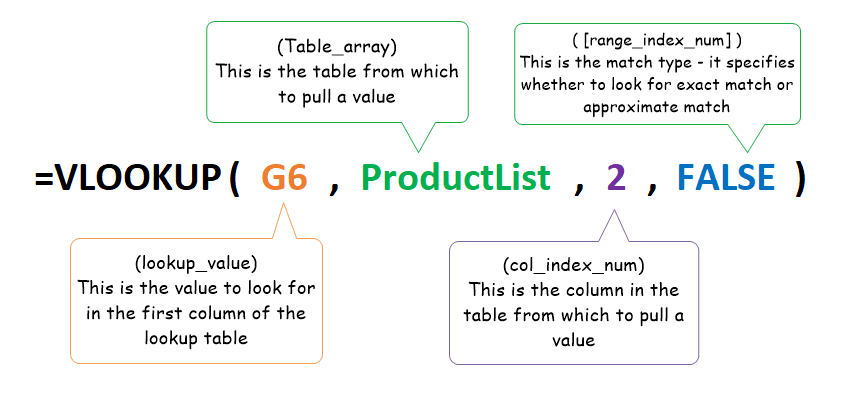The illustration below also shows the formula in the second cell of the price column:• The first arguments in the two formulas above are both referring to the same cell that contains the product ID we are looking for. (That is Cell G6).
• The second arguments are also referring to the same product list table from which to pull the product name and price respectively. (That is cell B4:D14 which is renamed to ProductList).
• The third arguments specify the columns in the table from which to pull the product name and price respectively. (That is column 2 for the product name, and column 3 for the price). This is the only formula that differs in the two versions of the VLOOKUP formulas.
• The fourth argument is the match type. Both formulas use the FALSE match type which means an exact match is needed.

As mentioned before, this is not a range lookup but an exact lookup.

Therefore, when a specified product ID doesn’t exist in the lookup table, the formula will result in an error, unlike the approximate match where the highest value that is lesser than the lookup value will be used.

All these cells encounter errors because the product ID cannot be found in the products list table.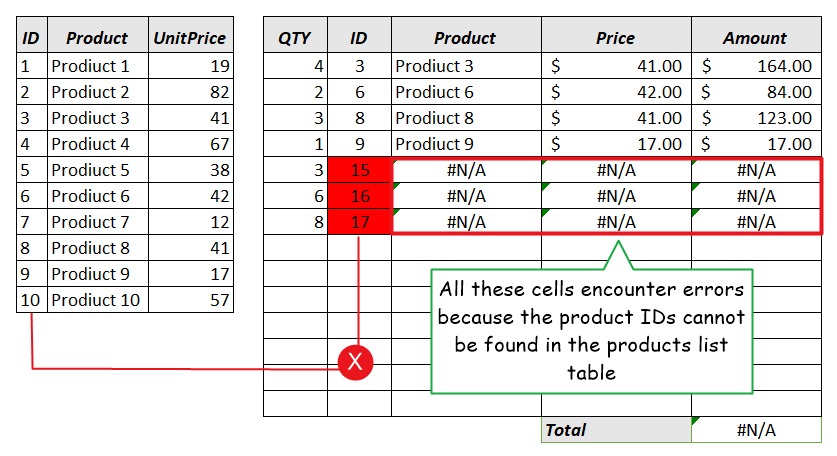How do you handle errors such as this one?

This task is qualified to make another example. So, let’s move on to the next.

## EXAMPLE 4: VLOOKUP if error Condition

As you can see in the above example, one of the causes of VLOOKUP not working is the fact that Excel is unable to find the value you are looking for.

This is the common cause. Though, not always. Sometimes the cause can be so obscure that you don’t know where to look.

It is true that the VLOOKUP function is powerful, but it isn’t flawless. Just like most good things, it too has its side effects.

This VLOOKUP example will help you to learn why the VLOOKUP value error occur when they do, and the techniques you can use to escape from these errors.

Know that: VLOOKUP value error is the kind of error that occurs when the lookup value is not found in the first column of the lookup table.

Using the worksheet in VLOOKUP example 3, let’s see how we can deal with the usual #N/A error.

See screenshot: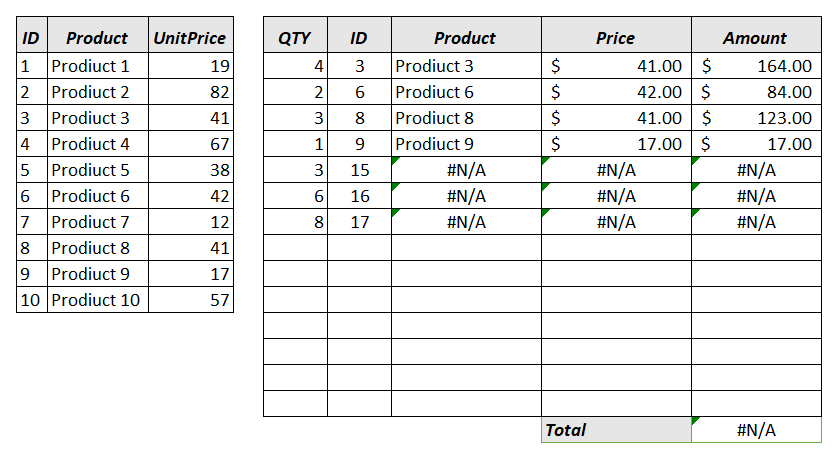In this worksheet, the cause of the error is apparent.

It is clear that the product IDs (15, 16 and 17) related to the error cells does not exist in the lookup table (or product list table).

If those products are new products in your list and have to be added, then the obvious solution to this value error is to include those products in the product list.

So, to do that, just expand the products list table by three rows and to the list the products IDs, names and their prices.

See screenshot:As soon as you add the missing products to the products lists, the invoice template works fine without errors.

See screenshot:This solution is indispensable if you must include the missing records to the lookup table. What if you mustn’t?

You might want Excel to do nothing or something else if it can’t find the search term.

Instead of the annoying #N/A error message, you can make Excel put a blank value in the cell, or return a cleaner message like a hyphen (-).

See screenshot:In the above illustration, when you enter a product that doesn’t exist in the products list table, the formula doesn’t encounter any error, unlike before where the #N/A error shows.

This problem is solved with the use of conditional logic to see whether a product ID is available or not. Some call this technique IF ERROR VLOOKUP.

The function used here is called the =IFERROR() function.

First of all, it checks the formula to see if there is an error in the results. This function will not execute VLOOKUP if error. It’ll only run the lookup formula if there is no error.

Below are the IF ERROR VLOOKUP formulas for the two columns:

For the product column

=IFERROR(VLOOKUP(G5,ProductList,2,FALSE),”-“)

The same formula in the first cell can be copied into each cell in the product column, provided you are using relative cell reference for the first argument (which is the lookup value)

For the price column

=IFERROR(VLOOKUP(G5,ProductList,3,FALSE),”-“)

Just like the product column, the same formula in the first cell can be copied into each cell in the price column using relative cell referencing for the lookup value.

See screenshot:The picture above is the worksheet in show formula mode that allows you to see all the worksheet formulas instead of the resulting values.

The main function of the IFERROR function in this formula is simple – it checks to see whether the VLOOKUP will generate any error. If the formula didn’t encounter any error, Excel runs the VLOOKUP smoothly. But if there really is an error, a hyphen is returned instead of #N/A.

See screenshot:## EXAMPLE 5: Finding a student’s score

The idea in this example and the next two will make more sense to you if you’re a student, a teacher, or have ever been one of these two.

In the table below contains a list of student’s names along with exam scores in various courses.

Let’s assume you want to return David’s Math score.The table is made up of five columns with the leftmost column being the names column and the scores of various courses in the remaining four columns.

Our aim here is to write a VLOOKUP formula that looks for David in the names column and retrieve his Math score.

The following worksheet illustrates this formula in action:### VLOOKUP Formula Analysis

Now, let’s have a bird’s-eye view of what really happened in the above worksheet.

See this first:There are four arguments in this VLOOKUP example.

• The first argument is cell The cell that contains the lookup value. AKA the value you are looking for in the leftmost column of the lookup table.
• The second argument is A1:E12. This is the range of cells from which to pull the student’s score. In other words, the lookup table.
• The third argument is number 4, the column index number. This is the column from which to pull the Math score. (That is column 4 of the lookup table).
• The final argument is set to 0 (the number zero) which stands for an exact match. This match type will search for the exact value as the lookup value. You can either use 0 (the number zero) or FALSE to show that you want an exact match.

VLOOKUP FORMULA FACTS: you can only ignore the final argument when you are working with a range lookup, where an approximate match is needed. By default, an approximate match is used when the argument is not specified. However, in case of VLOOKUP exact match, always remember to specify this argument, or you may get improper results.

In the merest form, let’s see how the formula retrieved the Math score of David.

First of all, it searches through the first column (or leftmost column) of the lookup table to find the name, David.

Once it spots the name in the list, it started counting to the right, up to the fourth column (which is the Math column), and retrieve the score from the cell that corresponds to the name David.

VLOOKUP FORMULA FACTS: By default, VLOOKUP can only look in the leftmost column of the lookup table. For instance, if you select a table and want to look in the second or third column, VLOOKUP cannot handle that. To avoid complications, always try and bring the lookup column first when preparing your data for VLOOKUP.

See screenshot:You can tweak the same formula to retrieve any student’s score for any course.

It’s just the matter of changing the column index number – 2 will retrieve the English score for the selected student, 3 will retrieve the French score, 4 will retrieve the Match score and 5 will also retrieve the Science score for the specified student.

For example, the formula below will retrieve the French score for Khan:

=VLOOKUP(G4,A1:E12,3,0)

The column index number has been changed from four to three. Since French is in the third column, and the lookup value in cell G4 is Khan, the formula will retrieve Khan’s French score.

See screenshot: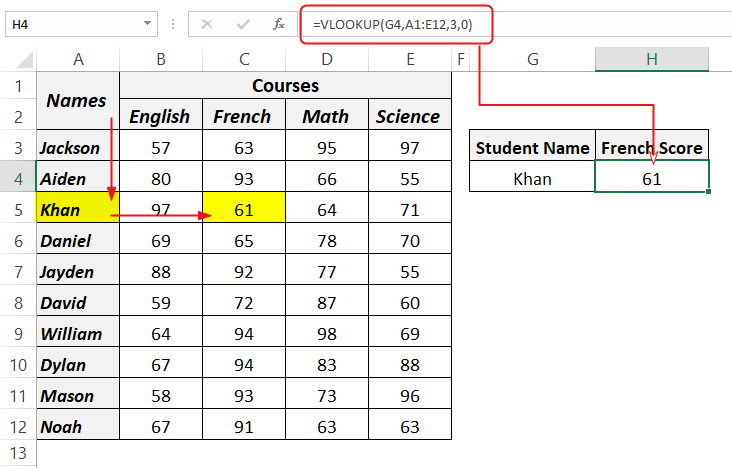As for the lookup value, to change a student name, just change the value in the cell containing the lookup value, and that is cell G4.

Now if you change cell G4 to any student’s name that exists in the list, the formula will adjust the result to that student’s score for French.

See screenshot:There’s a limitation to this worksheet though.

You can only change the student’s Name in cell G4 to retrieve his result, but in order to change his score for a different course, you must edit the formula to the respective column number.

What if there was a way the worksheet user can select the student name as well as the course name to retrieve a score without having to edit the formula again.

This is worth forming another example. To learn this trick, move on to the next example.

## VLOOKUP EXAMPLE 6: Two-way lookup

Two-way lookup is sometimes referred to as double lookup.

It is a formula that looks in both vertical and horizontal directions simultaneously, in order to find a cell where a specific column and row intersects.

There are two well know techniques to handle this request, one involves using VLOOKUP + MATCH and the other involves using INDEX+MATCH.

By default, the VLOOKUP function cannot handle this task alone, unless you employ a helper function which happens to be the MATCH function.

The purpose of the match function here is to give us the index number of the course we choose in the worksheet.

Let’s tweak the worksheet in the previous example for illustration.

See screenshot: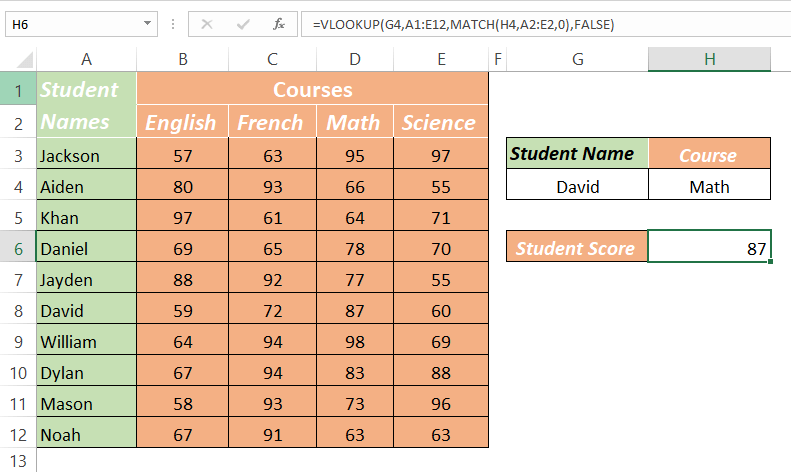With a little more effort, look at what we’ve got.

At the left-hand-side is the student exam score table, and to the right of the table is a tiny dashboard that allows the spreadsheet user to select a student name and course to retrieve scores based on the selected criteria.

The MATCH formula introduced here makes the formula so flexible that when the user changes the course, the formula automatically changes the column from which to retrieve the score.

### VLOOKUP formula analysis

The two-way lookup formula is stated below:

=VLOOKUP(G4,A1:E12,MATCH(H4,A2:E2,0),FALSE)

This formula has four arguments just like in the other examples. However, the only difference is that it has another function used as its third argument (col_index_num).

The MATCH function in the formula is used to supply a number to our VLOOKUP, the VLOOKUP then uses this number as its third argument (col_index_num).

For instance, if you run the MATCH function alone, it’ll give you a number indicating the position of the course you selected (AKA column number).

=MATCH(H4,A2:E2,0)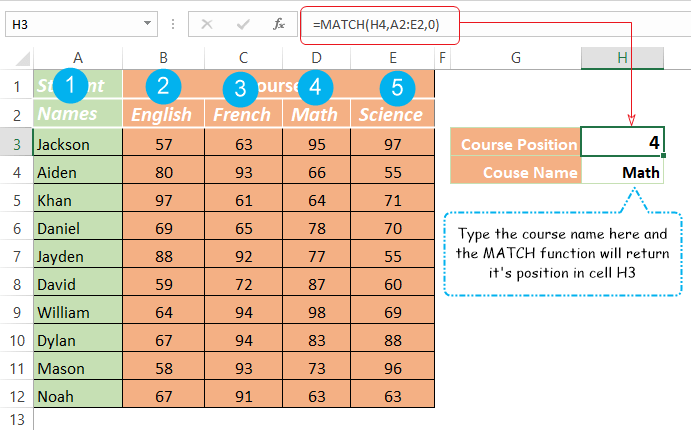As you can see here, the match function used the course name in cell H4 as the lookup value and return its position in range A2:E2 (the second row of the table).

If you type Math in cell H4, the formula will return 4 indicating that the scores for Math are located in the 4th column.

Thanks to the Match function, you can use the VLOOKUP formula to search the whole range of cells for the appropriate score.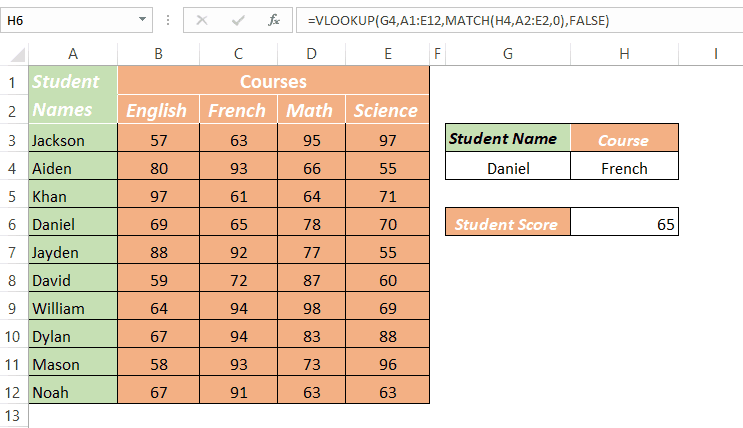To make it more interesting, you can use drop-down list to select the student’s name and their courses without manually typing them.

Let’s explore this technique in the next example.

## EXAMPLE 7: VLOOKUP with drop-down List

In the above example, to change the search terms (the name and the course), you’ll have to manually type them, but you can just as easily plug in the name and course using an In-Cell drop-down list.

Entering data manually increases your chances of typing wrong data into a formula’s input cell. The programmers will say “garbage in, garbage out”

And as a spreadsheet user, you should know better, that Excel formulas are only as good as the data they’re given.

Therefore, this VLOOKUP technique allows you or someone using your spreadsheet to click-in the student name and course, without typing them manually.

Below illustration is what I’m trying so hard to explain: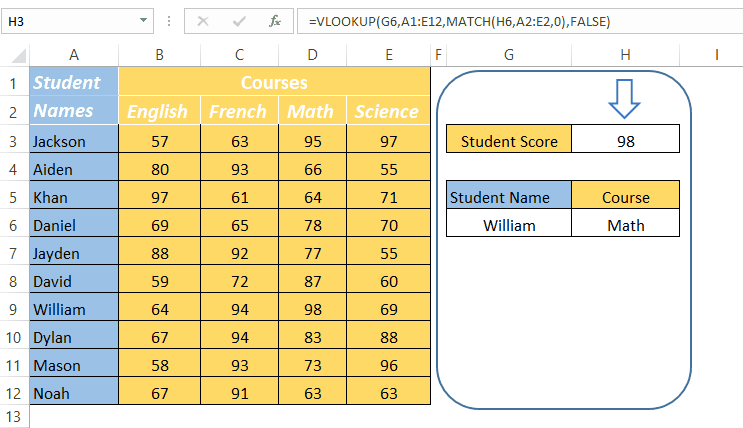Here, the formula used in the preceding example didn’t change at all.

The only thing you need to do here is to create a drop-down list for the cells containing the lookup values (cell G6 and H6).

Follow the following steps to learn how Excel drop down list is created:

• Click to activate the cell you wish to make the list for (Cell G6 and H6): Since we need two lists for two separate cells, you’ll have to create the lists one after the other.
• Go to Data →Data Tools→Data Validation: Under the Data tab, go to Data Tools category and click on Data Validation.• The Data Validation dialog appears with three tabs. Under the settings tab, there’s “validation criteria” called Allow, click the drop-down field below it and select list.
• Click in the field below the Source criteria and select the range that contains the list. (=\$A\$3:\$A\$12 for the names and =\$B\$2:\$E\$2 for the courses)

See screenshot: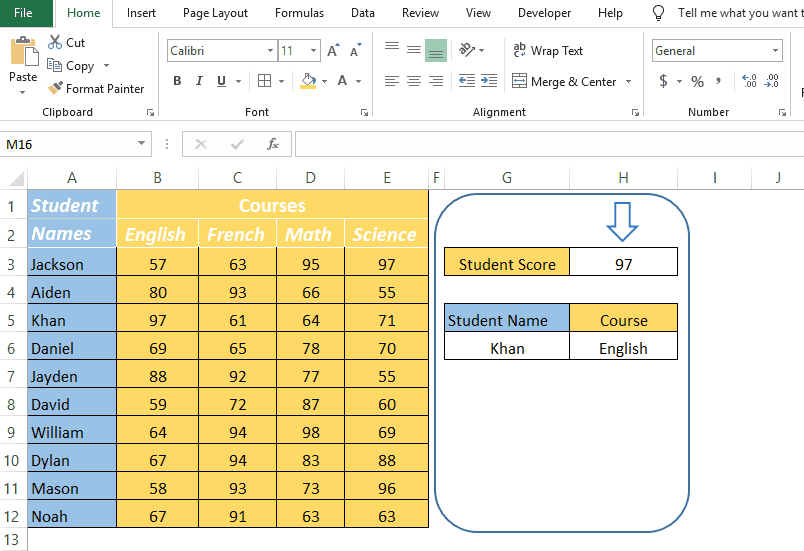After completing these steps for both cells, you’ll now be able to plug in the student’s name and their courses without typing them manually.

## EXAMPLE 8: VLOOKUP from another sheet

In this example, you’ll learn how to write the VLOOKUP formula to retrieve a value from a different sheet.

It is not always you’ll have your VLOOKUP table stationed in the same worksheet as the actual formula.

Sometimes the table through which you’ll look and retrieve values will be in a different worksheet.

Let’s take the following Excel invoice template for example. When you download and open the example file, you’ll see that one sheet (called price sheet) includes a product catalog, and the next one builds the invoice.

In this example, the invoice worksheet needs to use formulas that will refer to data in the previous sheet containing the product catalog.

See the invoice in the picture below: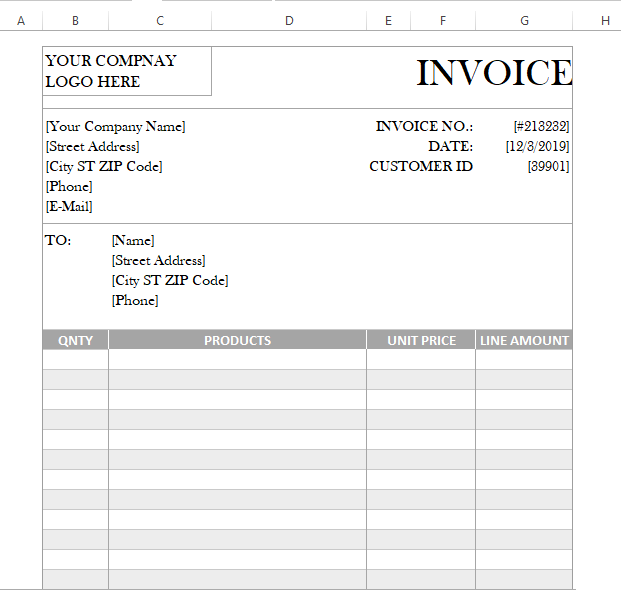This is a very clean invoice template worksheet. In VLOOKUP example 3, we have a similar invoice that retrieves products and prices from a different table, but in the same worksheet.

However, in this template, I want to teach you how to breathe life into a template like this one, by pulling product prices from a different sheet using THE VLOOKUP function.

If you haven’t yet, you should download the example file now to explore these multiple sheets VLOOKUP example.

Look in this example workbook, just after the example 8 worksheet is another worksheet named PriceList.

This sheet contains a table with two columns – products and price.

Below is a picture of the sheet containing the product catalog: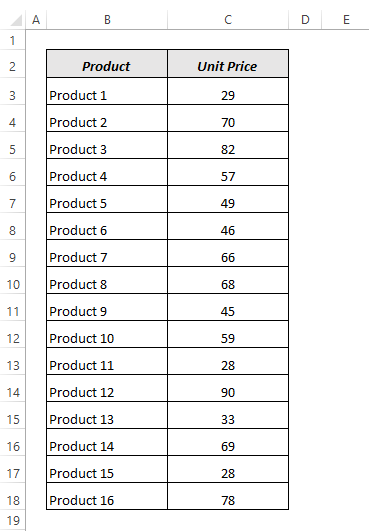Our goal in this example is to write a formula that performs VLOOKUP from another sheet – i.e. retrieving the products prices in the price column from a different sheet.

Here’s the formula that performs VLOOKUP between two sheets:

=VLOOKUP(C17,PriceSheet!B2:C18,2,FALSE)

See screenshot: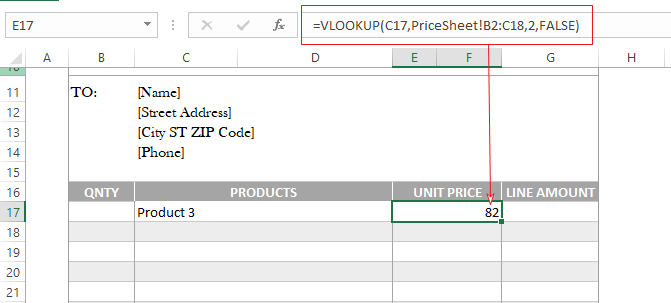### VLOOKUP formula analysis

Let’s break this formula into pieces and see what’s really happening.• C17: This cell contains the lookup value.
• PriceSheet!B2:C18: This is the lookup table located in a different sheet. The formula is using a table from a different sheet to retrieve the product price. There’s no mystery behind this technique: to do VLOOKUP from another sheet, in the second argument of the function, simply preface the range or table name with the worksheet name followed by an exclamation mark.
• 2: This is the column number in the table from which to retrieve the product price. The 2 in this argument will refer to the second column of the lookup table which is the price column.
• FALSE: This indicates that the formula should find a value that is an exact match as the lookup value in cell C17.

If you wish your worksheet to possess more functionality, you can use the drop-down list technique in the previous example for the product column.

This way, with just a couple of mouse clicks, you or your spreadsheet user can quickly fill your invoice with products whilst the VLOOKUP formula retrieves their prices.

See how awesome that is will be: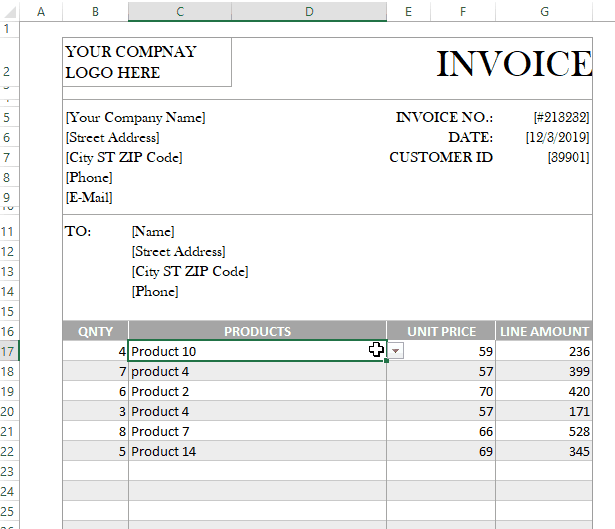Awesome huh!

Let’s continue to explore more in the next example.

## EXAMPLE 9: VLOOKUP from another Workbook

It is very common to perform VLOOKUP from another worksheet within the same workbook.

Yet, it is less common to perform VLOOKUP from another workbook.

The potential problem with this type of integration is that there’s no guarantee that the referenced workbook will always be available.

If you rename the referenced workbook, or move it to a different location, the link breaks.

However, Excel being so smart, doesn’t completely leave you stranded in this kind of situation. Instead, it continues to work with the most recent version of the data it was able to retrieve before the link broke. Lucky you!

In this example, you’ll learn how to write the VLOOKUP formula to retrieve a value from a different workbook.

Just like in the previous example, it is not always you’ll have your VLOOKUP table stationed in the same workbook.

Sometimes the table through which you’ll look and retrieve values will be in another workbook.

The concept to use here is almost the same as in the previous example.

Thus, to perform VLOOKUP from another workbook, when specifying the [table_array] argument, put the workbook name at the beginning of the reference, and then enclose it with square brackets. Then follow the file name with the sheet name, an exclamation mark, and then the range address or name.

If you really want to learn how this works, download the example file and create a new Excel workbook on your desktop.

Don’t change the default file name and worksheet name – leave it as Book1 (for the file name) and sheet1 (for the sheet name).

If you are using a different workbook for this example, then rename the file as Book1 and make sure it has a worksheet named sheet1.

If you are comfortable with this stuff and you know you won’t get confused, you can come along with your own workbook and worksheet names. It’ll work well provided you apply the necessary techniques.

Now that you have all this set, open the newly created workbook and in the sheet1 worksheet, create a product catalog like in the previous example.

You can also copy and past the table if you wish. However, ensure that you start pasting or creating your table from cell A1.

See screenshot:Now switch back to the example 9 worksheet, in the price column, type the formula below:

=VLOOKUP(C17,[Book1.xlsm]Sheet1!\$A\$1:\$B\$17,2,0)

The C17 in the formula is the lookup value which is referring to the content in Cell C7. Input any product name in this cell or you will get an error.

This VLOOKUP formula will retrieve the product price from the workbook called Book1 on your desktop.

Thus, if you want to VLOOKUP from another workbook, all you really need to do is write the workbook name in square brackets, and follow it with the sheet name, an exclamation mark and then the range.

However, if you don’t want to manually enter the [table_array] argument, there’s a simple technique to have Excel insert it for you.

First, open the workbook from which you want to reference. In our case, Book1 on the desktop.

Next, in the process of writing the formula, when you are about to specify the [table_array] argument, use Alt+Tab to navigate to Book1, then select the product catalog table or range.

When you do this look in the address bar and you’ll see that Excel has done the referencing for you, now provide the remaining arguments and press enter to execute the function.## VLOOKUP EXAMPLE 10: Partial text match with wildcard

Sometimes the content of your data can be so complicated that you don’t know exactly what you are looking for.

Take the following data for example; in the customer column, the customer names are prefixed with their account numbers.

Assuming you have only the customer names at hand, how will you search for a customer in this perspective?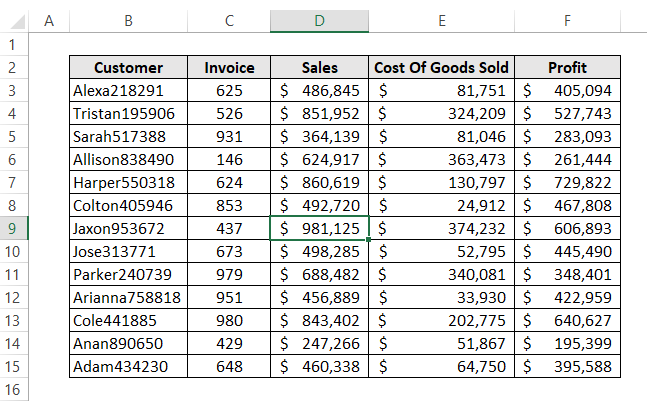In such cases, what you really need is a search tool that’s pliable enough to keep its eyes peeled for results that are similar but not exactly alike.

Power searchers will be happy to know that Excel’s wildcard characters make it possible to search for any part of a cell’s content.

For instance, in the above table, you can look up a customer by his name only without any worry about the account’s numbers.

There are three wildcards in excel used for advanced lookups. But in this example, let’s stick with the most popular one which is the asterisk (*) wildcard.

You can use the link below for a complete guide on this topic.

Related Link:  VLOOKUP wildcard – step by step guide.

With the above table, let’s say you want to retrieve the profit made on a customer named Harper, assuming you don’t know the number that appends his name.

Considering the following formula, let’s perform a normal VLOOKUP without the wildcard and see what result we will get.

=VLOOKUP(D3,B5:F18,5,FALSE)

See screenshot: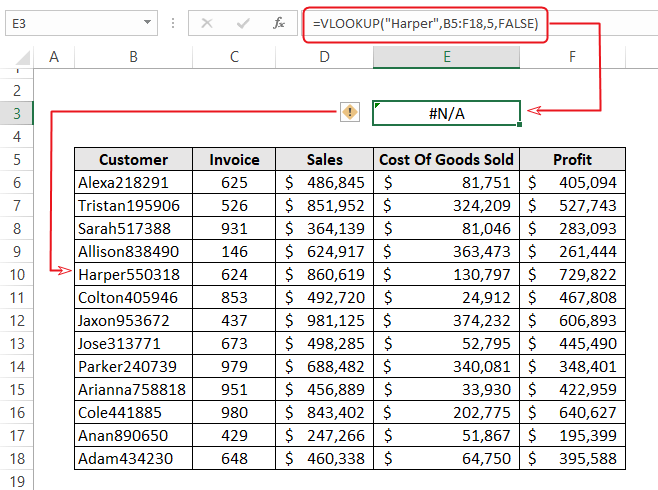Do you see?

What the formula tried to do is find a customer by name Harper, to retrieve the profit your company made with him.

Even though you do have an employee by that name, according to the data, the name doesn’t exist.

Excel is a very straight man, so you need to come straight when dealing with him.

Instead of just searching for Harper, use the asterisk wild card and search for Harper*.

Yes, that’s right! Append the name you wish to search with an asterisk symbol.

The formula should now look like this:

=VLOOKUP(“Harper*”,B5:F18,5,FALSE)

See the result now: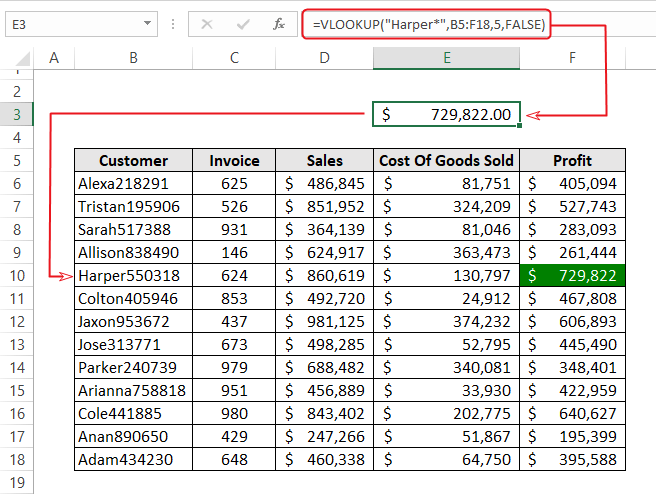Do you want to know how this happened?

Well, it’s simple.

The asterisk wildcard is used in the formula to replace all the numbers that come after the customer name – Harper.

The same way, if you want to search by the last numbers, just replace Harper with the asterisk wildcard (*550318), and excel will find the cell using the numbers only.

See screenshot: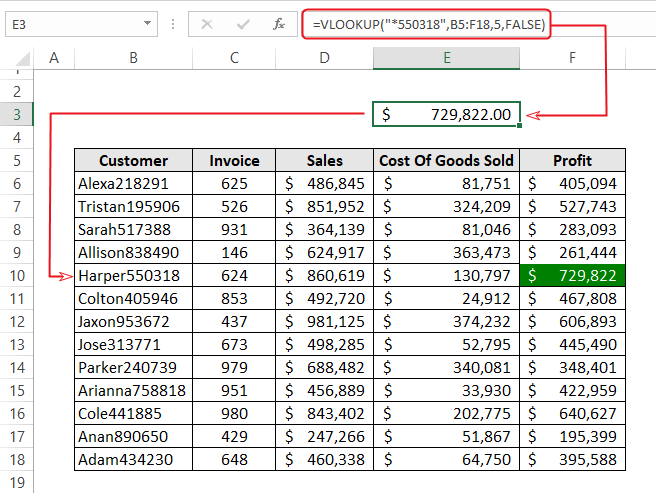In short, if you want to look for beginning characters, bring those characters before the asterisk (*). And if you want to look for ending characters, bring those characters after the asterisk (*).

In this example, the lookup values (customer name/number) are hard-coded in the formula, but you can just as easily retrieve information from other cells using the asterisk wildcard.

For example, if the customer name is in another cell, concatenate the cell address with an asterisk (*), and Excel will gladly execute your formula with the wildcard.

The following formula used the value in cell D3 as the lookup value and use the concatenate sign (&) to append the asterisk (*) wildcard.

=VLOOKUP(D3&”*”,B5:F18,5,FALSE)

See screenshot:Oh! I almost forgot.

You can also use dropdown list of the names to save you some time of typing.## EXAMPLE 11: VLOOKUP multiple criteria

In some cases, you may need to perform VLOOKUP if 2 criteria are met.

Unfortunately, this is one of the three most common VLOOKUP limitations.

Excel is designed in a way that it can only search through one column to retrieve a corresponding value when the search term is found.

Does that mean that the VLOOKUP function cannot lookup a value using multiple criteria?

Far from that.

With the technique used in this example, you can perform VLOOKUP with as many criteria as your data permits you.

Let’s consider the following example;This worksheet shows the dollar amount of sales made by sales representatives from January to April.

Imagine you want to get the sales Alexa makes in the month of March.

Alexa appears four times in the leftmost column of the table. If you use the VLOOKUP function to look for Alexa, the formula will spot the first Alexa appearance in the list.

Though, your intention is to spot the fourth Alexa appearance since it corresponds with the sales figure in March.

To skirt this shortfall of the function, you can use the helper column technique.

This technique will let you combine the two columns into a new column by concatenating them, then look into the combined column instead.

In this case, the combined column is now a helper column for you.

See screenshot:After combining the two columns in the helper column, you can then use the helper column as the lookup column to find any employees sales figure for any month.

For instance, if you want to find Alexa’s sales for March, use “Alexa|March” as your lookup value.

See the formula below:

=VLOOKUP(“Alexa|March”,D6:E25,2,FALSE)## Conclusion

Congratulations! You make it up to this point!

You can now shout on top of your voice and call yourself a VLOOKUP ninja. Trust me, it is a very big accomplishment.

You didn’t only learn the VLOOKUP function, you also learned several Excel tips and techniques that really worth its salt.

Explore this site for more in-depth Excel tutorials like this one.